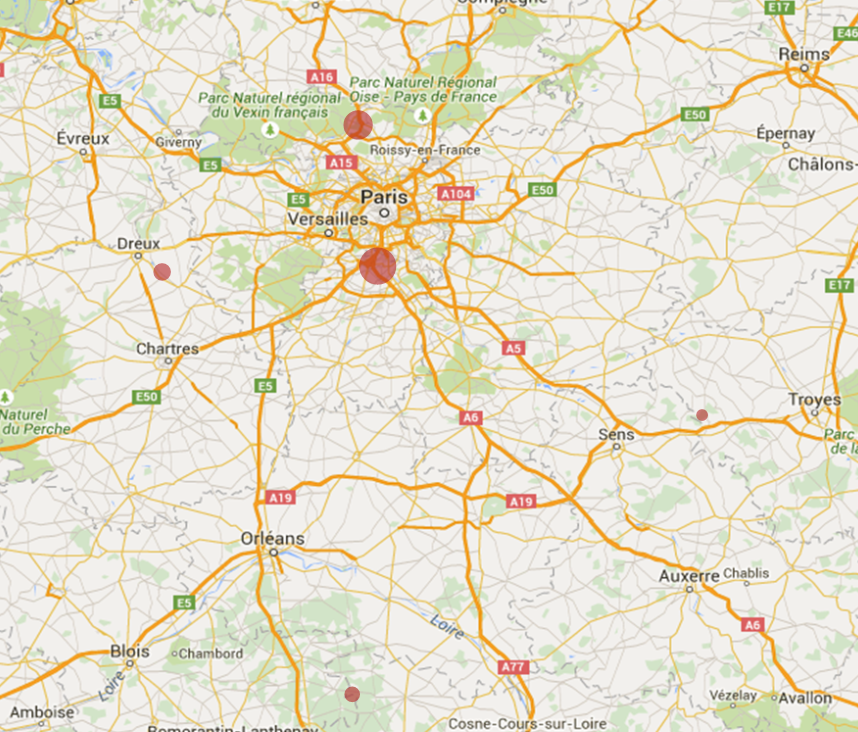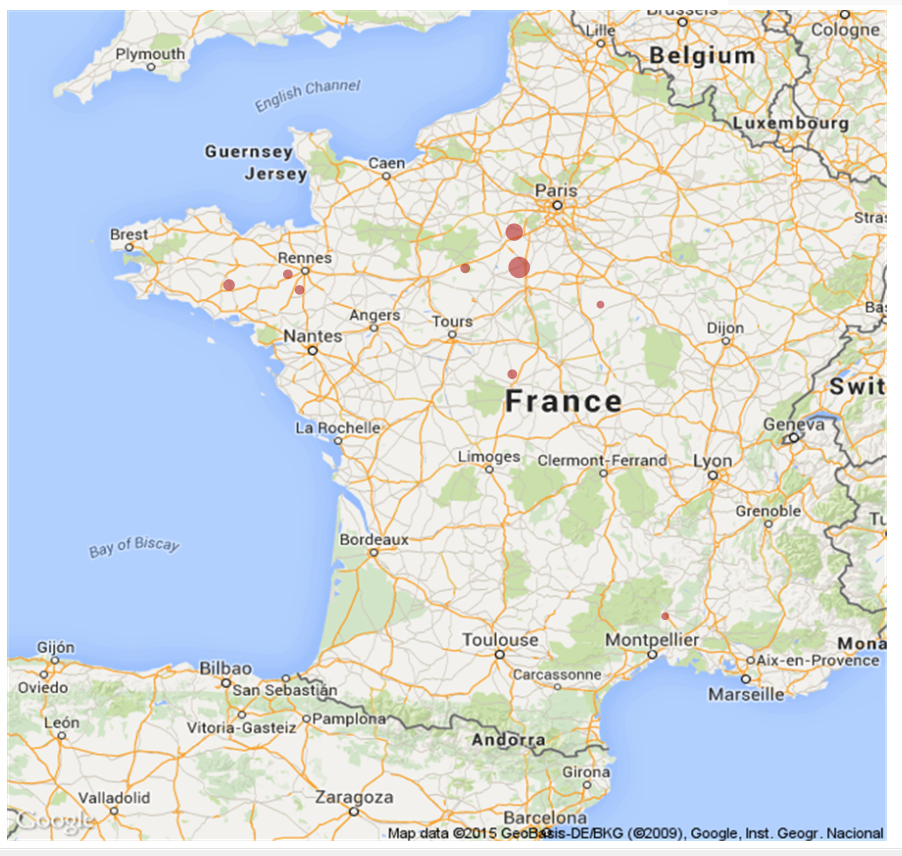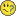QlikView App Development

Discussion Board for collaboration related to QlikView App Development.

HighlightedNot applicable

Hi All,

I have an offset on all the points drawed on the Google Map, but when i zoom in, the points are better placed but not in their place, anyone know why ?

Before : (wrong)Then, after zoom : (little bit better)I followed this tutorial :

Step-by-Step Google Map API v3 integration

Thank you,

Loup

Tags (2)
1 Solution

Accepted SolutionsMVP

Re: Google Map offset

 map_size_x1=       '900'; map_size_y1=     '850';

Try changing these to and see if that helps:

 map_size_x1= '640';

map_size_y1=     '400';

14 RepliesNot applicable

Re: Google Map offset

No one has had the same issue ?MVP

Re: Google Map offset

Do you have a legend or borders on your chart? Try clearing out everything from the chart area and see if that helps. Can you post a screenshot of the whole chart, including the borders?

Best,

SunnyNot applicable

Re: Google Map offset

I don't have any Legend on the chart...

Whole chart :The script :

max_zoom_level = 17; //maximum value 17

var_pi180=        '=pi()/180';

var_lat_offset1=    '0';

var_mc21=        '=256*pow(2,\$(var_zoom1))';

var_mc11=        '=256*pow(2,(\$(var_zoom1)-1))';

var_mid_lat1=    '=min([Latitude Assure])+(1+var_lat_offset)*((max([Latitude Assure])-min([Latitude Assure]))/2)';

var_mid_long1=    '=min([Longitude Assure])+(max([Longitude Assure])-min([Longitude Assure]))/2';

var_zoom1=        '=max(aggr(if(max( round(256*pow(2,(_zoom_level1 -1)))+( [Longitude Assure]  *((256*pow(2,_zoom_level1 ))/360)) )-min( round(256*pow(2,(_zoom_level1 -1)))+( [Longitude Assure]  *((256*pow(2,_zoom_level1 ))/360)) ) <map_size_x1 AND max((256*pow(2,(_zoom_level1-1)))+((0.5*log((1+(sin(([Latitude Assure])*pi()/180)))/(1-(sin(([Latitude Assure])*pi()/180)))))*((-256*pow(2,_zoom_level1))/(2*pi()))))-min((256*pow(2,(_zoom_level1-1)))+((0.5*log((1+(sin(([Latitude Assure])*pi()/180)))/(1-(sin(([Latitude Assure])*pi()/180)))))*((-256*pow(2,_zoom_level1))/(2*pi()))))<map_size_y1,_zoom_level1,1),_zoom_level1))';

//var_zoom=        '5';

var_maptype1=    '=if(isnull(only(maptype)),fieldvalue( '&chr(39)&'maptype'&chr(39)&', 1 ),maptype)';

map_size_x1=       '900';

map_size_y1=     '850';

SET HidePrefix='_' ;

// Field required for calcualting best zoom level

_zoom_level1:

Load RecNo( ) as _zoom_level1 autogenerate(max_zoom_level);

@

set hideprefix = 'maptype';

Thank youMVP

Re: Google Map offset

 map_size_x1=       '900'; map_size_y1=     '850';

Try changing these to and see if that helps:

 map_size_x1= '640';

map_size_y1=     '400';Not applicable

Re: Google Map offset

It is good !

So I have to change the size in order to keep this ratio ?

Thank you !

EDIT: Seems that I have to keep theses little dimensions...MVP

Re: Google Map offset

No problemJust in the script, I think. Front end object can stay and looks the same.

Best,

SunnyMVP

Re: Google Map offset

 EDIT: Seems that I have to keep theses little dimensions...

Not sure what dimensions you are talking about, can you please elaborate?Not applicable

Re: Google Map offset

Sorry, I was speaking about the size and not dimension (=in french).

If I keep the same front end object, it look horrible (in fact the map is extented), but when I change both size (script and front end), the offset appears, so I can't have a bigger mapMVP

Re: Google Map offset

Would you be able to share the application? I can take a look at it, if you want.

Best,

Sunny